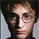# Fisher Transform Indicator by Ehlers

צפיות ‎4586‎
4586
Market prices do not have a Gaussian probability density function
as many traders think. Their probability curve is not bell-shaped.
But trader can create a nearly Gaussian PDF for prices by normalizing
them or creating a normalized indicator such as the relative strength
index and applying the Fisher transform . Such a transformed output
creates the peak swings as relatively rare events.
Fisher transform formula is: y = 0.5 * ln ((1+x)/(1-x))
The sharp turning points of these peak swings clearly and unambiguously
identify price reversals in a timely manner.
```////////////////////////////////////////////////////////////
//  Copyright by HPotter v1.0 01/07/2014
// 	Market prices do not have a Gaussian probability density function
// 	as many traders think. Their probability curve is not bell-shaped.
// 	But trader can create a nearly Gaussian PDF for prices by normalizing
// 	them or creating a normalized indicator such as the relative strength
// 	index and applying the Fisher transform. Such a transformed output
// 	creates the peak swings as relatively rare events.
// 	Fisher transform formula is: y = 0.5 * ln ((1+x)/(1-x))
// 	The sharp turning points of these peak swings clearly and unambiguously
// 	identify price reversals in a timely manner.
////////////////////////////////////////////////////////////
study(title="Fisher Transform Indicator by Ehlers", shorttitle="Fisher Transform Indicator by Ehlers")
Length = input(10, minval=1)
xHL2 = hl2
xMaxH = highest(xHL2, Length)
xMinL = lowest(xHL2,Length)
nValue1 = 0.33 * 2 * ((xHL2 - xMinL) / (xMaxH - xMinL) - 0.5) + 0.67 * nz(nValue1)
nValue2 = iff(nValue1 > .99,  .999,
iff(nValue1 < -.99, -.999, nValue1))
nFish = 0.5 * log((1 + nValue2) / (1 - nValue2)) + 0.5 * nz(nFish)
plot(nFish, color=green, title="Fisher")
plot(nz(nFish), color=red, title="Trigger")```
DONATE/TIP

BTC: 3FKWwtaYrf5NHZzaCi1fyAfQj7XSgtyCqejjcohen
It is not true. Look at 2013.10.30. We are have a high on the indicator = 6 and go to down, but the market move up. Also if you will be see this indicator in the realtime, as any other, it will not be show very good points to entry. In the hystory all indicators is good. You should know behavior of indicator for understanding how will move a market.
השב